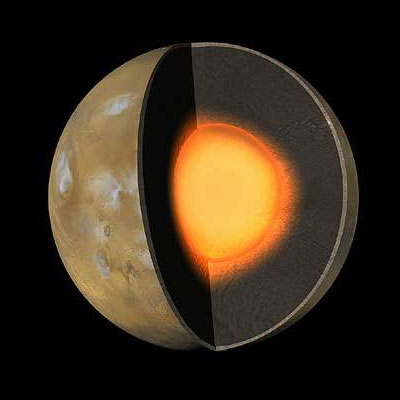Mars is ten times less massive than that of Earth. It is the second smallest planet in the Solar System. The mass of Mars is 7.08 x 1020 tons or 6.42 X 1020 metric tons. Mars total density is 3.933 grams/cubic centimeter. The diameter of Mars is 6,792 kilometers.

To illustrate these characteristics, Mars density is similar to that of the Earth’s moon. Mars is just 4/5 of the earth’s density.

Because Mars has less mass than Earth, the force of gravity on Mars is lesser as well. The gravity on Mars is 3/8 less than the force of gravity on earth. Thus something that would normally weight 100 pounds on earth would only weigh a measly 38 pounds on Mars. People who want to lose weight will have a jolly old time in Mars. Although it is important to note that weight is quite different from mass.

Mass is what makes up the object while weight is the force which gravity pulls you down towards the center of a planet. In this case, if you were to stand on Mars, you may weigh less but your body mass will remain the same.

The force of gravity on Mars is even smaller than it is on Mercury! Mercury is the smallest planet in the Solar System but it denser than Mars. Size and density is not interrelated in such a way that one would be affected by the other.

A Day on Mars – 1977